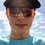# Smallest Possible Music Note + Planck Time

The Smallest music note you'll likely see on sheet music is the 16th note.2 connected 16th notes: Image

What if we went even further down the line of faster notes?

Planck time is defined as the time it takes for light to travel 1 Planck distance (L) or $≈ 5.4xE-44$ sec.There are more units of planck time in a second than there are seconds since the big bang: image

I'll assume one half note is equal to 1 second, and that each further note takes half the time to play as the last. With this in mind I

get the equation:

$\frac{1}{2^{x}} \ge 5.4xE-44$

$X ≈ 144$

That's a $\frac{1}{2^{145}}$th note. Imagine a note with 144 flags on it!!!a 64th note with 4 flags: image

We can go smaller and faster but it would make no difference since the human brain won't be able to determine beats faster than around a 32nd note.Note by Brack Harmon
2 years, 11 months ago

This discussion board is a place to discuss our Daily Challenges and the math and science related to those challenges. Explanations are more than just a solution — they should explain the steps and thinking strategies that you used to obtain the solution. Comments should further the discussion of math and science.

When posting on Brilliant:

• Use the emojis to react to an explanation, whether you're congratulating a job well done , or just really confused .
• Ask specific questions about the challenge or the steps in somebody's explanation. Well-posed questions can add a lot to the discussion, but posting "I don't understand!" doesn't help anyone.
• Try to contribute something new to the discussion, whether it is an extension, generalization or other idea related to the challenge.

MarkdownAppears as
*italics* or _italics_ italics
**bold** or __bold__ bold
- bulleted- list
• bulleted
• list
1. numbered2. list
1. numbered
2. list
Note: you must add a full line of space before and after lists for them to show up correctly
paragraph 1paragraph 2

paragraph 1

paragraph 2

[example link](https://brilliant.org)example link
> This is a quote
This is a quote
    # I indented these lines
# 4 spaces, and now they show
# up as a code block.

print "hello world"
# I indented these lines
# 4 spaces, and now they show
# up as a code block.

print "hello world"
MathAppears as
Remember to wrap math in $$ ... $$ or $ ... $ to ensure proper formatting.
2 \times 3 $2 \times 3$
2^{34} $2^{34}$
a_{i-1} $a_{i-1}$
\frac{2}{3} $\frac{2}{3}$
\sqrt{2} $\sqrt{2}$
\sum_{i=1}^3 $\sum_{i=1}^3$
\sin \theta $\sin \theta$
\boxed{123} $\boxed{123}$

Sort by:

For a sixteenth note, there are many periods of sinusoidal vibration of the air within that single note. Presumably, the shortest possible note would have to be multiple planck lengths long, in order to allow for some sort of vibration. Interesting idea.

- 2 years, 11 months ago